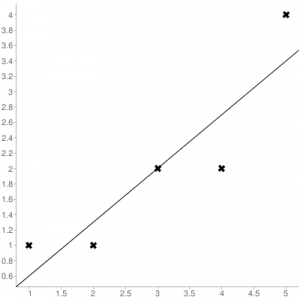# Python | Mean Squared Error

The Mean Squared Error (MSE) or Mean Squared Deviation (MSD) of an estimator measures the average of error squares i.e. the average squared difference between the estimated values and true value. It is a risk function, corresponding to the expected value of the squared error loss. It is always non – negative and values close to zero are better. The MSE is the second moment of the error (about the origin) and thus incorporates both the variance of the estimator and its bias.

Steps to find the MSE

1. Find the equation for the regression line.

(1)2. Insert X values in the equation found in step 1 in order to get the respective Y values i.e.

(2)3. Now subtract the new Y values (i.e.) from the original Y values. Thus, found values are the error terms. It is also known as the vertical distance of the given point from the regression line.

(3)4. Square the errors found in step 3.

(4)5. Sum up all the squares.

(5)6. Divide the value found in step 5 by the total number of observations.

(6)Example:
Consider the given data points: (1,1), (2,1), (3,2), (4,2), (5,4)
You can use this online calculator to find the regression equation / line.Regression line equation: Y = 0.7X – 0.1

X Y1 1 0.6
2 1 1.29
3 2 1.99
4 2 2.69
5 4 3.4

Now, using formula found for MSE in step 6 above, we can get MSE = 0.21606

MSE using scikit – learn:

 from sklearn.metrics import mean_squared_error     # Given values  Y_true = [1,1,2,2,4]  # Y_true = Y (original values)     # calculated values  Y_pred = [0.6,1.29,1.99,2.69,3.4]  # Y_pred = Y'     # Calculation of Mean Squared Error (MSE)  mean_squared_error(Y_true,Y_pred)

Output: 0.21606

MSE using Numpy module:

 import numpy as np     # Given values  Y_true = [1,1,2,2,4]  # Y_true = Y (original values)     # Calculated values  Y_pred = [0.6,1.29,1.99,2.69,3.4]  # Y_pred = Y'     # Mean Squared Error  MSE = np.square(np.subtract(Y_true,Y_pred)).mean()

Output: 0.21606

Attention reader! Don’t stop learning now. Get hold of all the important CS Theory concepts for SDE interviews with the CS Theory Course at a student-friendly price and become industry ready.

My Personal Notes arrow_drop_upPossess good Mathematical and Statistical Foundation Data Science Enthusiast Addicted to Python

If you like GeeksforGeeks and would like to contribute, you can also write an article using contribute.geeksforgeeks.org or mail your article to contribute@geeksforgeeks.org. See your article appearing on the GeeksforGeeks main page and help other Geeks.

Please Improve this article if you find anything incorrect by clicking on the "Improve Article" button below.